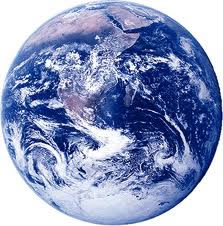OCR GCSE - Forces B

Page 1

Springs and Stretching, Momentum, Free Body Diagrams, Balanced Forces, Stopping Distances

A long wire is stretched using a large force. The table shows the length of the wire for different forces.

 Force (N) Length (m) 0 ? 100 10.0 200 10.8 300 11.6 400 12.4 500 13.2 600 14.5 700 16.01. What was the original length of the wire, when no force is applied?

2. What is the extension of the wire when the first 100N is applied?

3. This wire shows 'linear' extension properties up to a point.

What is the maximum length of the wire before it becomes 'non-linear'? (The limit of proportionality')

4. What is the spring constant for this wire for small forces applied?

• A) 10 N/m
• B) 10.9 N/m
• C) 100 N/m
• D) 125 N/m

5. What force would produce a length of 11.2 m?

• A) 125 N
• B) 225 N
• C) 250 N
• D) 275 N

6. The formula given below is used to calculate the energy stored in a stretched wire or spring:

Energy = 0.5 x (spring constant) x (extension)2

What is the energy stored in the stretched wire at a length of 10.0m?

• A) 40 J
• B) 50 J
• C) 400 J
• D) 500 J

7. Which of these does not show linear extension properties?

• A) Rubber bands
• B) Metal Wires
• C) Springs
• D) Steel cables

8. As the Earth orbits the Sun, which measurement is constantly changing?

• A). The size of the force causing circular motion
• B). The velocity of the Earth
• C). The speed of the Earth
• D). The distance between the Earth and the Sun9. A remote controlled car is switched on and accelerates from rest. Which of these 4 free body diagrams correctly shows the relative sizes of the forces acting on the car?10. The car in question 9 is hard to accelerate or decelerate if the mass is larger. This is called the car's

• A). inertia
• B). kinetic energy
• C). potential energy
• D). resistance

11. As the car accelerates, a strong wind also pushes on the car as shown in this diagram showing the view from above the car:Which of the following arrows shows the NET force (resultant force) acting on the car?12. To increase the momentum of a toy car, which two quantities must be increased?

 1st Quantity 2nd quantity A. mass velocity B. weight acceleration C. acceleration mass D. mass force